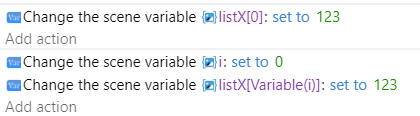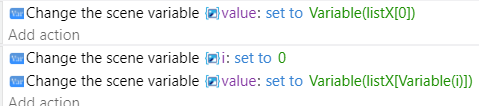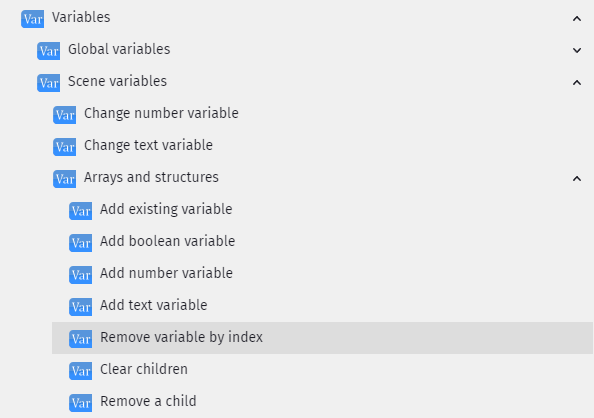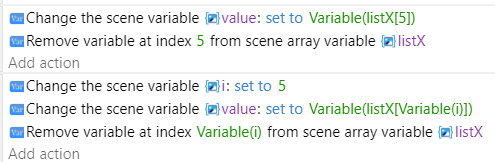# How do I access a Specific Child Array

Im confused about how arrays exactly work in Gdevelop

I figured out how to store a player’s x and y points for every time i move

I’m trying to access a specific stored point but i don’t know how to access that number

the “YE” was just a random test can someone help to where it will display the value of the tenth child or recorded point ig

Thank youArray (index starts at 0)

1 Like

can u explain? a little better I dont really understand what that means

You acces it using ArrayName[index of child]

To set a value you use the variable name followed by the child or index within brackets.
You can use a number or a number variable.To get the value, you do the same thing in reverseNote: using the new variable method, Variable() isn’t needed as long as you add the variable to the setup screen. IE declare the variable.

You can use a repeat to go through the array using another variable, be sure to set the i variable to 0 first. This would set each index to the value of the variable i. meaning the value would be equal to its index.

Under the same plus symbol is for each child variable this works for arrays and structures
This would go through the array and put the value into the variable child and the index into index
(all variable names can be anything, it’s best to declare them in the variable screen, the last box child name is optional)

In this example, it goes through the entire array, it puts the value into the variable child and the index (starting at zero) into the variable Index. It then sets the variable at index using the variable named Index to the current value (child) times 2.

It can be confusing but it gets easier.

There are other array actionsYou can remove an index by a number or a number variable. Any other indexes would move up to fill the spot. Meaning if there were indexes 0 thru 9 and you remove #5 then 6-9 would move up and it the array would be 0-8.

This would remove the index at 5. It sets the current value to the variable value first.remove a child is for structures
clear children removes all of the children for structures or arrays (indexes)